Test: Probability and Statistics - 2

# Test: Probability and Statistics - 2

Test Description

## 20 Questions MCQ Test Topic-wise Tests & Solved Examples for IIT JAM Mathematics | Test: Probability and Statistics - 2

Test: Probability and Statistics - 2 for Mathematics 2023 is part of Topic-wise Tests & Solved Examples for IIT JAM Mathematics preparation. The Test: Probability and Statistics - 2 questions and answers have been prepared according to the Mathematics exam syllabus.The Test: Probability and Statistics - 2 MCQs are made for Mathematics 2023 Exam. Find important definitions, questions, notes, meanings, examples, exercises, MCQs and online tests for Test: Probability and Statistics - 2 below.
Solutions of Test: Probability and Statistics - 2 questions in English are available as part of our Topic-wise Tests & Solved Examples for IIT JAM Mathematics for Mathematics & Test: Probability and Statistics - 2 solutions in Hindi for Topic-wise Tests & Solved Examples for IIT JAM Mathematics course. Download more important topics, notes, lectures and mock test series for Mathematics Exam by signing up for free. Attempt Test: Probability and Statistics - 2 | 20 questions in 60 minutes | Mock test for Mathematics preparation | Free important questions MCQ to study Topic-wise Tests & Solved Examples for IIT JAM Mathematics for Mathematics Exam | Download free PDF with solutions
 1 Crore+ students have signed up on EduRev. Have you?
Test: Probability and Statistics - 2 - Question 1

### A problem is given to three students A,B and C whose chances of solving it are 1/2 ,1/3 and 1/4 respectively. The probability that this problem will be solved, is :

Detailed Solution for Test: Probability and Statistics - 2 - Question 1

Required probability = 1 - probability that no student solve the problem.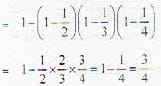Test: Probability and Statistics - 2 - Question 2

### 6 boys and 6 girls sit in a row randomly. The probability that all the girls sit together is :

Detailed Solution for Test: Probability and Statistics - 2 - Question 2

Required probability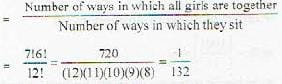Test: Probability and Statistics - 2 - Question 3

### Probabilities of three students A, B and C to pass an examination arc respectively 1/3,1/4 and 1/5. The probability that exactly one student will pass is :

Detailed Solution for Test: Probability and Statistics - 2 - Question 3

Required probability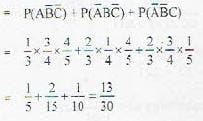Test: Probability and Statistics - 2 - Question 4

Different words are written with the letters of PEACE. The probability that both E’s come together is :

Detailed Solution for Test: Probability and Statistics - 2 - Question 4

Required probability =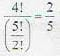Test: Probability and Statistics - 2 - Question 5

The probability of throwing 6 at least one in four throws of a die is :

Detailed Solution for Test: Probability and Statistics - 2 - Question 5

Probability of throwing 6 at least once = 1 - probability of not throwing 6.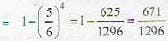Test: Probability and Statistics - 2 - Question 6

An untrue coin is such that, when it is tossed the chances of appearing head is twice the chances of appearance of tail. The chance of getting head in one toss of the coin is :

Detailed Solution for Test: Probability and Statistics - 2 - Question 6

If k is probability of occurrence of head., then k/2 will be probability of occurence of tail, so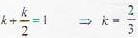Test: Probability and Statistics - 2 - Question 7

The probability of randomly choosing 3 defectless bulbs from 15 electric bulbs of which 5 bulbs are defective, is :

Detailed Solution for Test: Probability and Statistics - 2 - Question 7

Probability required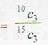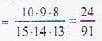Test: Probability and Statistics - 2 - Question 8

Probability of four digit numbers, which are divisible by three, formed out of digits 1,2,3,4.5 is :

Detailed Solution for Test: Probability and Statistics - 2 - Question 8

Four digit numbers formed with digits 1,2 .4 ,5 will be divisible by three, so required probability is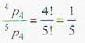Test: Probability and Statistics - 2 - Question 9

If X is uniformly distributed over (0, 10), the probability that 1 < X < 6 is

Detailed Solution for Test: Probability and Statistics - 2 - Question 9

Probability density function of X which is uniformly distributed in (0, 10) is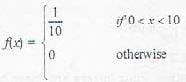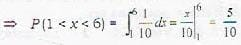Test: Probability and Statistics - 2 - Question 10

The choice of throwing 12 in a single throw with three dice is

Detailed Solution for Test: Probability and Statistics - 2 - Question 10

If the numbers appearing on the face of dice are such that the sum of the values on their faces lesser than 6 is equal to 6, with the constraint that 6 is not subtracted from a single dice. So, required probability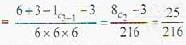Test: Probability and Statistics - 2 - Question 11

X is a continuous random variable with probability’ function f(x) = N exp(-x2+6x) - ∝ < x < ∝, the value of N is

Detailed Solution for Test: Probability and Statistics - 2 - Question 11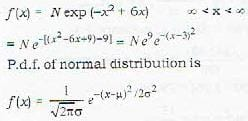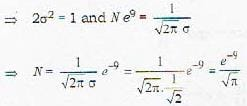Test: Probability and Statistics - 2 - Question 12

X is an exponential random variable with parameter λ with p.d.f. (x) = λe-7x if x ≥ 0 = 0 if x < 0, identify the correct one

Detailed Solution for Test: Probability and Statistics - 2 - Question 12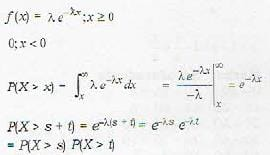Test: Probability and Statistics - 2 - Question 13

Determine the value of K for which the function given by f [x, y) = kxy for x = 1, 2, 3 and y = 1,2, 3 can serve as a joint probability distribution

Detailed Solution for Test: Probability and Statistics - 2 - Question 13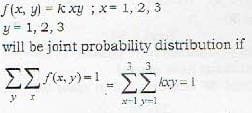⇒ K(1 + 2 + 3) (1 + 2 + 3) = 1 ⇒ k = 1/36

Test: Probability and Statistics - 2 - Question 14

On your way to work, you have to drive through a busy juction where you may be stopped at traffic lights. The cycle of the traffic light is 2 minutes of green followed by 3 minutes of red. What is the expected delay in the journey, if you arrive at the junction at a random time uniformly distributed over the whole 5 minute cycle is?

Detailed Solution for Test: Probability and Statistics - 2 - Question 14

Delay in journey is denoted by x then its probability density function.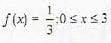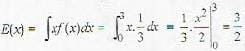Test: Probability and Statistics - 2 - Question 15

Let X have the poission distribution with parameter λ such that P(X = k - 1) = r(k) P(X = K). Then r[K] is

Detailed Solution for Test: Probability and Statistics - 2 - Question 15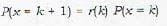⇒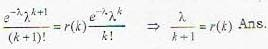Test: Probability and Statistics - 2 - Question 16

If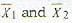are the means of two distributions such that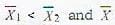is the mean of the combined distribution, then

Detailed Solution for Test: Probability and Statistics - 2 - Question 16

If nelements has mean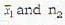elements has mean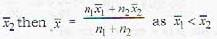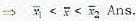Test: Probability and Statistics - 2 - Question 17

Suppose that the expected number of accidents per week at an industrial plant is 4. The number of workers injured in each accident is independent random variable with a common mean of 2. Assume also that the number of workers injured in each accident is independent of the number of accidents that occur. The expected number of injuries during a week is

Detailed Solution for Test: Probability and Statistics - 2 - Question 17

E(xy) = E(x) E(Y) = 4 x 2 = 8

Test: Probability and Statistics - 2 - Question 18

Let X and Y be independent random variables μand μy and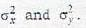Then Var (XY) equals

Detailed Solution for Test: Probability and Statistics - 2 - Question 18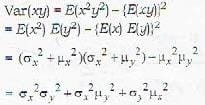Test: Probability and Statistics - 2 - Question 19

f(x) = k, exp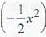for all x ∈ R; can be a probability density function for

Detailed Solution for Test: Probability and Statistics - 2 - Question 19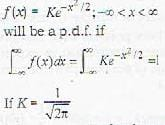it becomes standard normal distribution.

Test: Probability and Statistics - 2 - Question 20

Let x and y be independent random variables with binomial distribution B(10, 1 /3) and B(20, 1/3) respectively. E[x + y] is

Detailed Solution for Test: Probability and Statistics - 2 - Question 20

E (x + y) = E (x) + E(y)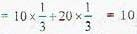Expectation of binomial distribution is np if B(n, p) is the binomial distribution.

## Topic-wise Tests & Solved Examples for IIT JAM Mathematics

27 docs|150 tests
 Use Code STAYHOME200 and get INR 200 additional OFF Use Coupon Code
Information about Test: Probability and Statistics - 2 Page
In this test you can find the Exam questions for Test: Probability and Statistics - 2 solved & explained in the simplest way possible. Besides giving Questions and answers for Test: Probability and Statistics - 2, EduRev gives you an ample number of Online tests for practice

## Topic-wise Tests & Solved Examples for IIT JAM Mathematics

27 docs|150 tests